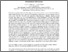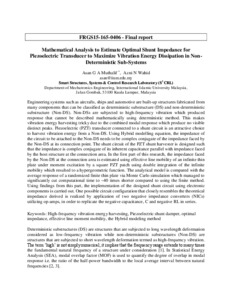# FRGS15-165-0406: MATHEMATICAL ANALYSIS TO ESTIMATE OPTIMAL SHUNT IMPEDANCE FOR PIEZOELECTRIC TRANSDUCER TO MAXIMIZE VIBRATION ENERGY DISSIPATION IN NON-DETERMINISTIC SUB-SYSTEMS

Muthalif, Asan G. A. (2018) FRGS15-165-0406: MATHEMATICAL ANALYSIS TO ESTIMATE OPTIMAL SHUNT IMPEDANCE FOR PIEZOELECTRIC TRANSDUCER TO MAXIMIZE VIBRATION ENERGY DISSIPATION IN NON-DETERMINISTIC SUB-SYSTEMS. Research Report. UNSPECIFIED. (Unpublished)Preview
PDF

## Abstract

Engineering systems such as aircrafts, ships and automotive are built-up structures fabricated from many components that can be classified as deterministic substructure (DS) and non-deterministic substructure (Non-DS). Non-DSs are subjected to high-frequency vibration which produced response that cannot be described mathematically using deterministic method. This makes vibration energy harvesting tricky due to the combined modal response which produce no visible distinct peaks. Piezoelectric (PZT) transducer connected to a shunt circuit is an attractive choice to harvest vibration energy from a Non-DS. Using Hybrid modelling equation, the impedance of the circuit to be attached to the Non-DS needs to be complex conjugate of the impedance faced by the Non-DS at its connection point. The shunt circuit of the PZT shunt harvester is designed such that the impedance is complex conjugate of its inherent capacitance parallel with impedance faced by the host structure at the connection area. In the first part of this research, the impedance faced by the Non-DS at the connection area is estimated using effective line mobility of an infinite thin plate under moment excitation by a square PZT patch using double integration of the infinite mobility which resulted to a hypergeometric function. The analytical model is compared with the average response of a randomized finite thin plate via Monte Carlo simulation which managed to significantly cut computational time to ~40 times shorter compared to using the finite method. Using findings from this part, the implementation of the designed shunt circuit using electronic components is carried out. One possible circuit configuration that closely resembles the theoretical impedance derived is realized by application of two negative impedance converters (NICs) utilizing op-amps, in order to replicate the negative capacitance, C and negative RL in series.

Item Type: Monograph (Research Report) T Technology > TA Engineering (General). Civil engineering (General) > TA349 Mechanics of engineering. Applied mechanics Kulliyyah of Engineering > Department of Mechatronics Engineering Ir. Ts. Dr Asan Gani Abdul Muthalif 30 Mar 2021 10:22 30 Mar 2021 10:22 http://irep.iium.edu.my/id/eprint/65614View Item